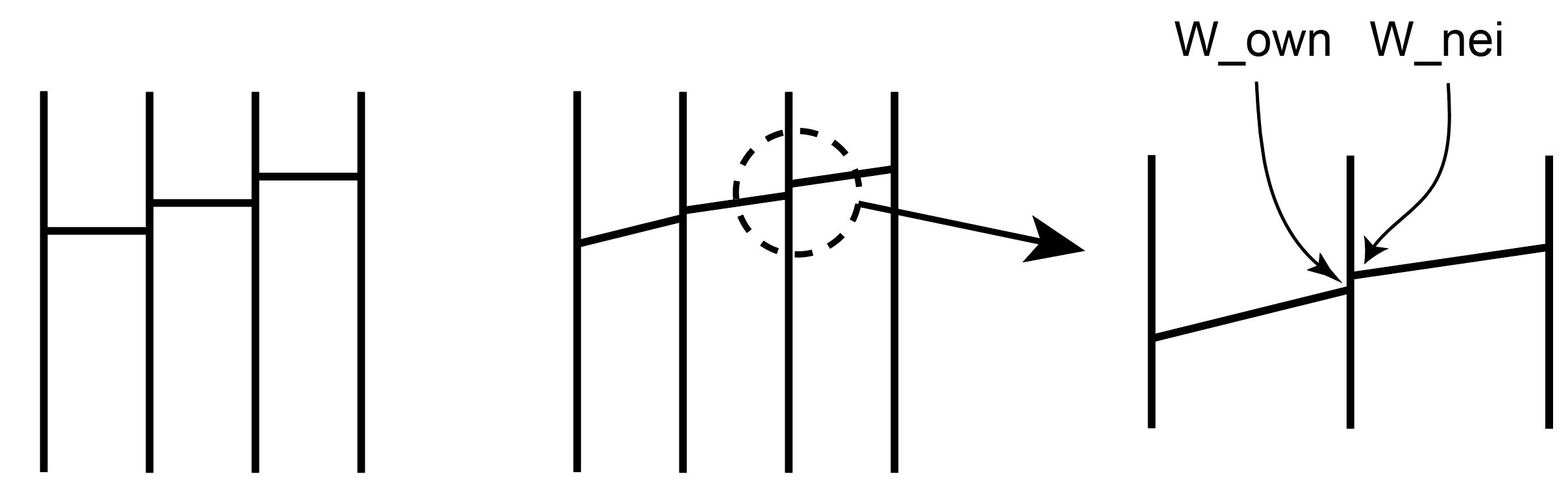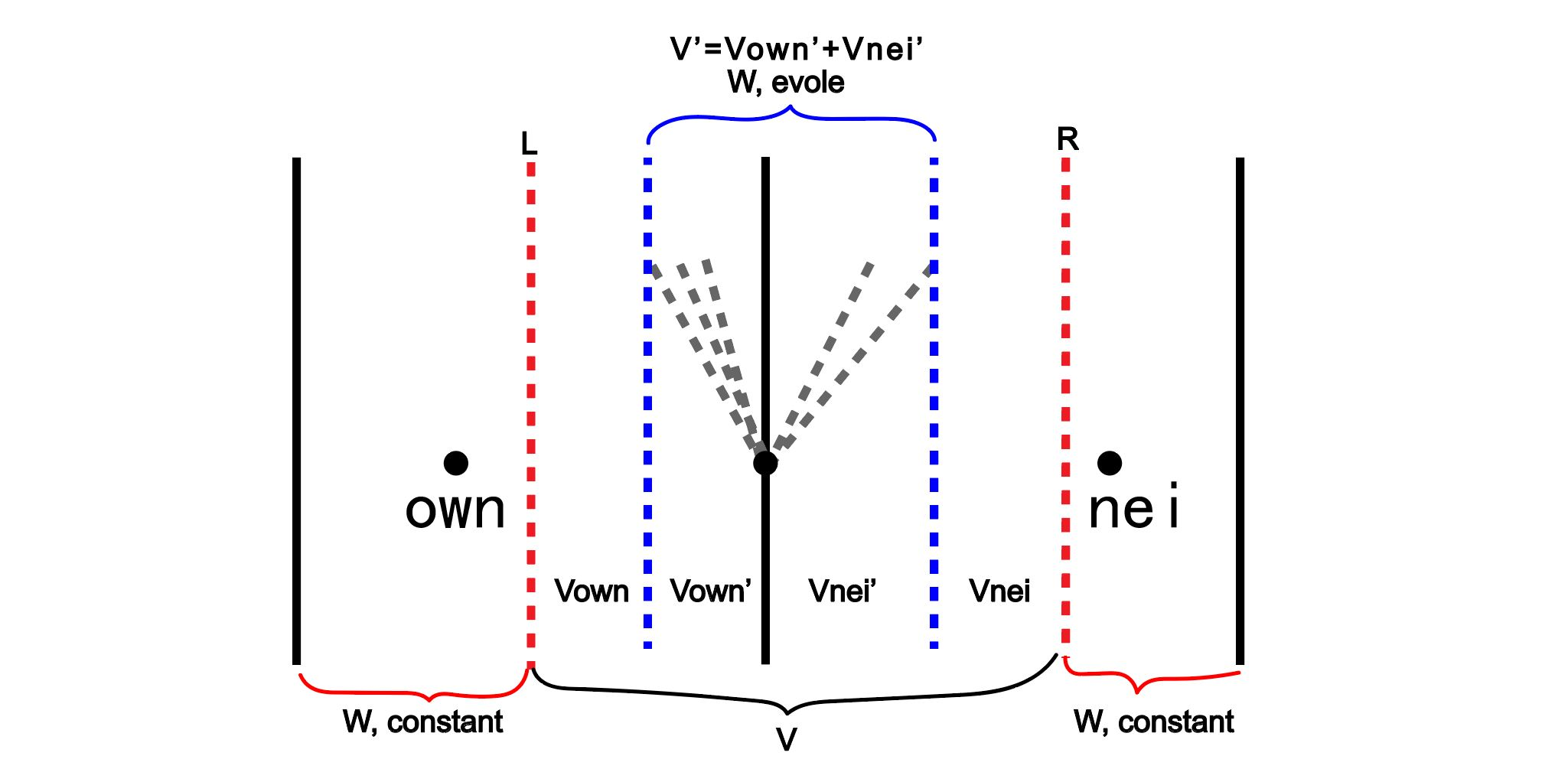# CFD中的跨音速通量格式

## HLL格式

own网格与nei网格：在有限体积法中，可以将网格面毗连的网格单元定义为这个面的own单元以及nei单元。每个内部面均存在own单元与nei单元。1) 网格值重组示意图

(1)$\int_{V}\bfW^{t+\dt}\rd V= \int_{V}\bfW^t\rd V +\int_t^{t+\dt}F(\bfW_L)\rd t - \int_t^{t+\dt}F(\bfW_R)\rd t$

(2)$\int_{V}\bfW^{t+\dt}\rd V= \bfW^t V +\bigl(F(\bfW_L^t) - F(\bfW_R^t)\bigr)\dt$

(3)$\bfW^t V\approx\bfW_{own}^t (V_{own}+ V_{own}')+\bfW_{nei}^t (V_{nei}+ V_{nei}')$

(4)$\int_{V}\bfW^{t+\dt}\rd V= \bfW_{own}^t (V_{own}+ V_{own}')+\bfW_{nei}^t (V_{nei}+ V_{nei}') +\bigl(F(\bfW_L^t) - F(\bfW_R^t)\bigr)\dt$2) 网格示意图

(5)$\int_{V}\bfW^{t+\dt}\rd V= \int_{V_{own}}\bfW^{t+\dt}\rd V +\int_{V'}\bfW^{t+\dt}\rd V +\int_{V_{nei}}\bfW^{t+\dt}\rd V$

(6)$\int_{V}\bfW^{t+\dt}\rd V= V_{own}\bfW^{t}_{own} +\int_{V'}\bfW^{t+\dt}\rd V +V_{nei}\bfW^{t}_{nei}$

(7)$\int_{V'}\bfW^{t+\dt}\rd V = \bfW_{own}^t V_{own}'+\bfW_{nei}^t V_{nei}' +\bigl(F(\bfW_L^t) - F(\bfW_R^t)\bigr)\dt$

(8)$\frac{1}{V'}\int_{V'}\bfW^{t+\dt}\rd V = \Bigl(\bfW_{own}^t V_{own}'+\bfW_{nei}^t V_{nei}' +\bigl(F(\bfW_L^t) - F(\bfW_R^t)\bigr)\dt\Bigr)\frac{1}{V'}$

(9)$V_{nei}'\approx\Delta t|\bfS_f| a_{nei}^f, V_{own}'\approx\Delta t|\bfS_f| a_{own}^f$

(10)$F(\bfW_R^t)\approx\phi_{nei}\bfW_{nei}^t,F(\bfW_L^t)\approx\phi_{own}\bfW_{own}^t$

$$\frac{1}{V'}\int_{V'}\bfW^{t+\dt}\rd V$$可以理解为$$V'$$控制体的平均的$$\bfW$$的值。在HLL方法中，$$\bfW$$被认为是$$\bfW_{hll}$$

(11)$\bfW_{hll} = \frac{\bfW_{own}^t |\bfS_f| a_{own}^f+\bfW_{nei}^t |\bfS_f| a_{nei}^f +\bigl(\phi_{own}\bfW_{own}^t - \phi_{nei}\bfW_{nei}^t\bigr)}{|\bfS_f| a_{nei}^f+|\bfS_f| a_{own}^f}$

$$V'$$控制体的任一点的值均为$$\bfW_{hll}$$，因此面上的值为$$\bfW_{hll}$$。需要注意的是，方程(11)定义的是黎曼问题部分向左或者部分向右传递的情况。如果特征值均向左或者向右传递，HLL降低为普通插值格式。

## 矢通量分裂（vanLeer、AUSM）

(12)$M^+=\frac{1}{4}(M+1)^2,M^-=-\frac{1}{4}(M-1)^2$

• $$M_f\geqslant 1: F(\bfW)_f=F(\bfW^{+})_f$$

• $$M_f\leqslant -1: F(\bfW)_f=F(\bfW^{-})_f$$

• $$-1<M_f<1: F(\bfW)_f=F(\bfW^{+})_f+F(\bfW^{-})_f$$

• 质量通量：$$F(\bfW_1)_f=\phi_f\rho_f c_f M_f$$;

• 动量通量：$$F(\bfW_2)_f=\phi_f\rho_f c_f^2 (M_f^2+\frac{1}{\gamma_f})$$;

• 能量通量：$$F(\bfW_3)=\phi_f\rho_f c_f^3 M_f (\frac{1}{2}M_f^2+\frac{1}{\gamma_f-1})$$;

(13)$F(\bfW_1)_f=\phi_f\rho_f c_f M_f=\phi_f\rho_f c_f\frac{1}{4}(M_f+1)^2-\phi_f\rho_f c_f\frac{1}{4}(-M_f+1)^2$

(14)$\begin{split} F(\bfW_2)_f=\phi_f\rho_f c_f \Bigl(\frac{1}{4}(M_f+1)^2\Bigr)\left((\gamma_f-1)M_fc_f+2c_f\right)\frac{1}{\gamma_f} \\ +\phi_f\rho_f c_f \Bigl(\frac{1}{4}(-M_f+1)^2\Bigr)\left((\gamma_f-1)M_fc_f+2c_f\right)\frac{1}{\gamma_f} \end{split}$

(15)$\begin{split} F(\bfW_3)_f=\phi_f\rho_f c_f \Bigl(\frac{1}{4}(M_f+1)^2\Bigr)\left((\gamma_f-1)M_fc_f+2c_f\right)^2\frac{1}{2\gamma_f^2-2} \\ +\phi_f\rho_f c_f \Bigl(\frac{1}{4}(-M_f+1)^2\Bigr)\left((\gamma_f-1)M_fc_f+2c_f\right)^2\frac{1}{2\gamma_f^2-2} \end{split}$

(16)$\begin{split} F(\bfW_1)_f=\phi_f^+\rho_f^+c_f^+M_f^+&+\phi_f^-\rho_f^-c_f^-M_f^- = \\ &\Bigl(\phi_f^+\rho_f^{+}c_f^{+}\frac{1}{4}(M_f^{+}+1)^2\Bigr)+\Bigl(-\phi_f^-\rho_f^{-}c_f^{-}\frac{1}{4}(M_f^{-}-1)^2\Bigr) \end{split}$

AUSM格式与vanLeer格式的主要区别，在于AUSM格式认为方程变量中的对流与压力来自于不同的贡献，因此在处理通量的情况下， 需要将对流贡献与压力贡献单独处理。将压力贡献提取出来之后，回看一维欧拉方程的通量形式：

• 质量通量对流贡献：$$F(\bfW_1)_f=\phi_f\rho_f c_f M_f$$;

• 动量通量的对流贡献以及压力贡献之和：$$F(\bfW_2)_f=\phi_f\rho_f c_f^2 M_f^2+\phi_fp$$;

• 能量通量对流贡献：$$F(\bfW_3)=\phi_f\rho_f c_f M_f H_f$$;

(17)$p^+=\frac{1}{2}p(1+M),p^-=\frac{1}{2}p(1-M)$

(18)$p^+=\frac{1}{4}p(M+1)^2(2-M),p^-=\frac{1}{4}p(M-1)^2(2+M)$

(19)$\phi_fp=\phi_f^+p^+_f+\phi_f^-p^-_f= \frac{1}{2}\phi_f^+p^+_f(1+M^+_f) + \frac{1}{2}\phi_f^-p^-_f(1-M^-_f)$